Performance Improvement Of Smith Predictor Through Automatic Computation Of Dead Time

VERONESI Massimiliano1

It is known that the classical tuning formula for typical Proportional- Integral-Derivative (PID) controllers in general provides unsatisfactory results for industrial plants where the time delay exceeds the dominant lag time. For this reason, alternative strategies have been studied in order to cope with this problem and, in this context, the most popular scheme is the Smith Predictor. In this paper the theory behind this algorithm is explained and its implementation through YS170 controller language and CENTUM CS 3000 Control Drawing Builder are presented in order to verify their effectiveness in industrial environments. This approach requires a good model of the process under control. In fact, the performance of the Smith Predictor can decrease dramatically (become unstable) due to modelling errors, especially for the dead time which, contrary to what would be expected, can vary considerably depending on the working conditions (i.e. the fluid flow). In this paper a simple adaptive law for the automatic tuning of the model time delay is suggested. When this method is applied, the performance of the Smith Predictor is easily improved due to the automatically tuned model dead time and the control algorithm is capable of meeting variable working conditions.

1. Yokogawa Italia, Industrial Automation Department

INTRODUCTION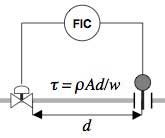Figure 1 Time Delay Due to the Fluid Transfer Time in a Pipeline

In process control it is not uncommon for systems to be affected by dead times, due to material transfer times. This is evident typically in pipelines. A fluid of density ρ and flow rate w in a section of pipe A the length of d takes the amount of time τ = ρAd/w to cover the whole distance (see Figure 1).

This means the controller (human or digital) can only be aware in retrospect of the effect of actions taken, and decisions must therefore be based on previous situations, typically resulting in troublesomely long oscillations around the steady state value. In this case, however, we can take some countermeasures which are not so complicated and do not stray far from the traditional and popular PID control architectures.

Switching to mathematical relationships, a first order SISO (Single Input Single Output) process affected by dead time can be described by a differential equation such as

x(t)=f(x(t);u(t-τ) (1)

where x(t) is the measured variable at time t, u(t) is the manipulated variable and τ is the dead time.

Considering the simpler and more popular linear case, (1) can be re-written as

x(t) = ax(t) + bu(t-τ) (2)

which, by Laplace transformation, corresponds to the usual algebraic relationship in the complex variable "s"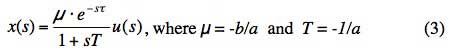Such an equation is used to describe all those processes with dynamic behaviour that are dominated by a one time constant T and a time delay τ (FOPDT: First Order Plus Time Delay).

Referring to the simple PID formula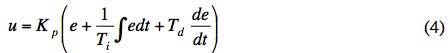where u is a manipulated variable, and e is an error (given by the difference between a setpoint value and a measured variable), the transfer function of the PID is:

RPID (s) = Kp (1 + 1/sTi + sTd) (5)

From the previous equations it is clear that the control action is computed as the sum of three terms and that, through the tuning of Kp, Ti and Td it is possible to give different weights to them in order to achieve the desired performance for a closed loop system. Proportional action has exactly the same trend in error; the integral action (proportional to the sum of the past errors) is used to reset the steady state error; and the derivative action (proportional to the error tendencies) can ultimately anticipate the future error behaviour during the transient. This last effect of the derivative action, however, can not be useful if the process is affected by dead time. Its action, in fact, is based on the evaluation of something that has already started to happen. In this case, the process variable starts to change after τ seconds and so the derivative of the error remains zero throughout the duration of the dead time. In other words the measured value does not contain adequate information to foresee the future.

The usual PID controllers are suitable for controlling stable process in which the τ/T ratio is small. In fact, if it is large, tuning the PID parameters would be difficult, and lengthy trial-and-error activities could cause heavy loads for the plants, possibly exposing them to danger. Moreover, disregarding this heavy load, the final results would not be adequately satisfactory.

THE SMITH PREDICTOR

A simple algorithm for control processes affected by dead time is the one proposed by Hägglund (named Predictor-PI)2 and based on the idea of decreasing the manipulated variable by an amount equal to all that was computed in the last τ seconds. However the more popular scheme for control processes affected by time delay was proposed by O.J.M. Smith6 and is shown in Figure 2.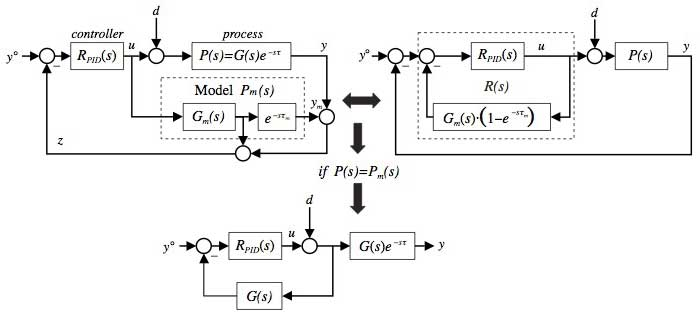Figure 2 Equivalent Schemes for the Smith Predictor

Let P(s) = G(s)e-sτ be the transfer function of the process and let's indicate the setpoint with y° and a generic load disturbance with d.

This algorithm requires a minimal knowledge of the process to describe it through a transfer function (model)

Pm(s) = Gm(s)e-sτm (6)

where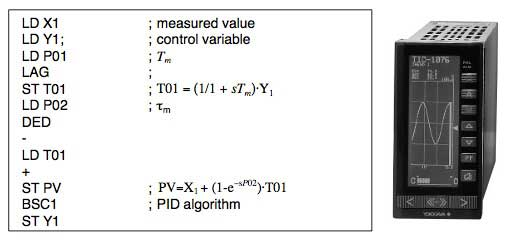Figure 3 Smith Predictor Realised through the YS170 Programming Language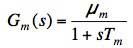is a rational function of the complex variable "s", which should approximate the process without delay. The parameters of the model can be achieved by popular identification experiences such as the one based on the open loop system response to a step change of the control variable.

As shown in Figure 2, the feedback is closed not on the process value y, but on the z variable, which has the same value that y had τm seconds earlier; and therefore it is in some ways a "prediction" of the measure; that is why this control architecture is called Smith "Predictor".

So, the resulting controller transfer function is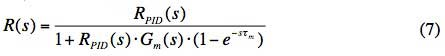The poles of the model are zeros of R(s) and so, if the model is a good approximation of the real process, the controller can perform a zero/pole cancellation independently by the values of the PID parameters.

In fact, being D(s) the Laplace transformation of the load disturbance, the following transfer functions can be computed as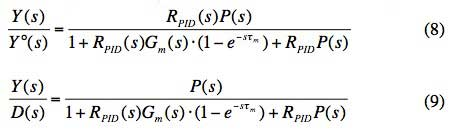and so, if τm = τ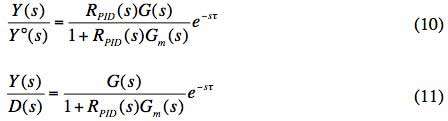Therefore, from a theoretical point of view, and with a good model (Gm(s) = G(s)), the PID controller can be tuned as it would be for a process without a time delay; thereby making it easy to achieve its best performance.

Thanks to digital technologies, it is not so difficult to realise a Smith Predictor algorithm. Modern controllers have a rich library of parameters and function blocks with which it is easy to build a transfer function; among them there are, for instance, the ones corresponding to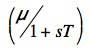and e-sτ

operators.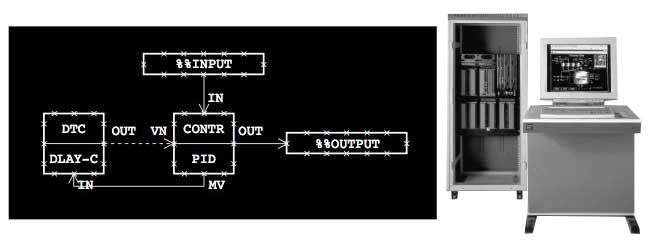Figure 4 Smith Predictor Designed with the Control Drawing Builder of CS1000/3000 Yokogawa DCS

In Figures 3 and 4, two examples of the Smith Predictor are shown. In the first one, the low level YS170 language was used, while in the second one the algorithm is performed by the function blocks available in the CENTUM CS1000/3000 control drawing builder. The DLAY-C block performs the transfer function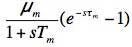and its output is added to the error internally at the PID block (through the parameter VN). For this reason the Compensation Gain (CK) of the PID block has to be set to -1. The meanings of the other variables are explained in the figures themselves. In both cases the PID algorithm is performed by a single action (BSC for the YS170 and PID for CENTUM), as dictated by the programming language.

Specifically, in the PID block the INPUT COMPENSATION option has to be selected in the Function Block Detail Builder / Control Calculation Tab. Then the dead time is computed as the product of the parameter SMPL (available by DLAY-C tuning window) and the number of sample points which has to be set by engineers in the Function Block Detail Builder / Basic Tab.

It must be emphasized that in case the process has an integrator (that is s=0 is a pole for the transfer function G(s)), the Smith Predictor is not able to accommodate a load disturbance D(s) on the process input. In fact, it can be proved that in a steady state the ratio between the process value and the load disturbance is proportional to the model gain and time delay. This means that the integral of the load disturbance will not be compensated; or in other words, that the controller is not able to reset the steady state error.

The most effective scheme for load disturbance compensation is the one proposed by Matausek, Micic3. The idea is to eliminate additional feedback by subtracting from the manipulated variable an amount proportional to the difference between the process variable and its estimation, as provided by the model. That difference, in fact, is by construction the best approximation of the load disturbance that we have after the dead time.

AUTOMATIC DETERMINATION OF THE DEAD TIME VALUE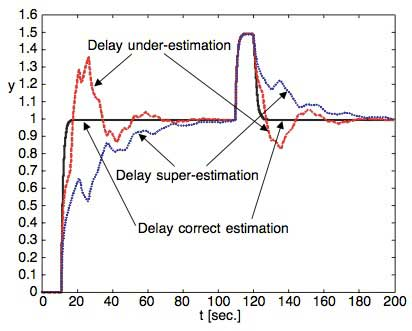Figure 5 Ideal Trend Compared to the One Achievable with ± 50% Delay Estimation Mistake

The performance of the Smith Predictor dramatically decreases (become unstable) due to modelling errors, especially for the dead time (τm). This is very dangerous because it can vary widely depending on the working conditions (i.e. the fluid flow).

Let's consider, for instance, a simple FOPDT process with T=1 sec. and τ=10T. A step change in the setpoint is applied and then, when τ =100 sec, a load disturbance will be simulated. In Figure 5 the trends achieved with ± 50% delay estimation mistakes are illustrated. The performances (achieved by re-tuning proportional gain and integral time) are worse than that of the ideal situation in which τ =τm. In fact, in case of under-estimation, the PID algorithm will anticipate the action generating oscillation around the steady state value. However, on the other hand, in case of super-estimation, the PID will be late and the approach to setpoint will therefore be slow and hesitating.

If it is not possible to measure the flow and thereby dynamically adapt the delay time value τmin the algorithm, a filter on the modelling error (y-ym) could be a countermeasure, but its robustness is a drawback to the speed, especially for load disturbance rejection. Therefore it can be useful to find a way to autonomously adjust τm to τ. For such an achievement, the following adaptive law can be proposed: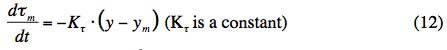that is, starting from τom,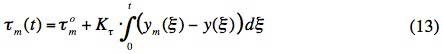It is not difficult to understand the reasoning behind this formula.

In fact, if τom< τ, during the transient between y1° and y2° > y1°, the model response is faster than the process one, hence ym- y > 0 for the most part of the time. Therefore ,τm being proportional to that difference, it will grow from the initial value τom to τ . On the other hand, if τom > τ, the model response will be slower than the process one and so ym- y < 0 for an extensive period. Thus, due to the proposed adaptive law, τmwill decrease. Ultimately, when ym= y, the derivative of τmwill become stable. Analog consideration holds in the case of y2° > y1°.

If Gm(s) = G(s), by indicating with ŷ the process variable not affected by delay, it holds that

y(t) = ŷ (t - τ)
ym(t) = ŷ (t - τom) (14)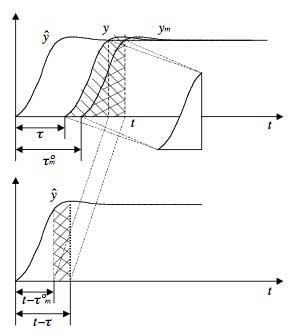Figure 6 Graphical Representation of Equation (15)

Looking at Figure 6, if τom> τ, it can be seen that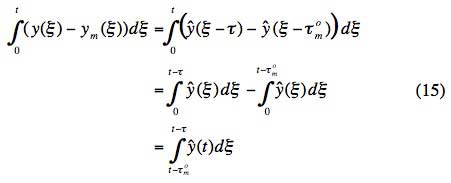Then, considering a simple FOPDT process, the open loop response without dead time is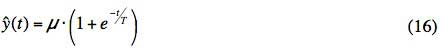Therefore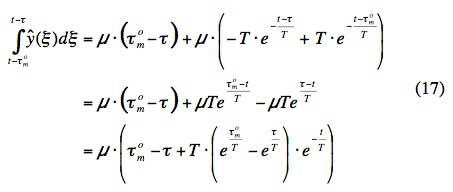This is the proof that the proposed adaptation law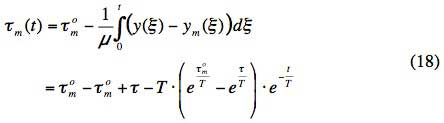brings τmto τ for t → ∞ faster the more they are different at the beginning.

The algorithm works even if the loop is closed and the feedback allows the use of a coefficient Kτ1/μ in order to accelerate its convergence. In this way the loop can be left in Automatic Mode and the algorithm will autonomously identify the value of τ through a few simple setpoint changes.

Some countermeasures have to be considered because the sign of Kτ must agree with that of the setpoint change, and the adaptive law has to be disabled when the process variable joins the steady state. In fact, after that, a load disturbance would be misunderstood as a change of the manipulated variable and so would provide a change in τm. In order to avoid these behaviours, the following formula (suggested in [Veronesi, Visioli, - 2000])8 9 can be used: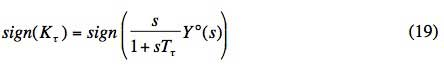In this manner, the time delay is quickly identified and therefore the proposed algorithm can improve the robustness of the Smith Predictor in control processes affected by long time delays. The effectiveness of the method is shown in Figure 7, where some setpoint step changes are applied. It is easy to see that the performance is dramatically better than the one achievable without model delay adaptation and that a higher value of Kτ can speed up the computation of τm.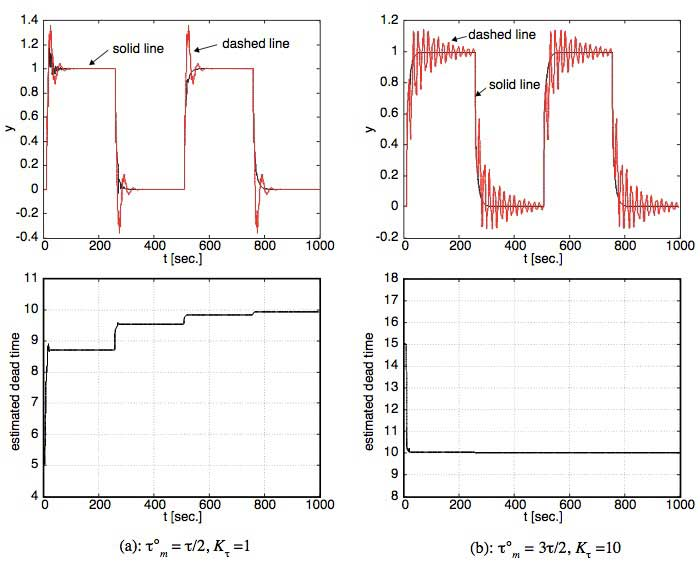Figure 7 Comparison Between a Smith Predictor with Model Delay Automatic Determination (solid line) and a Simple Smith Predictor (dashed line)

The proposed technique is also robust enough to accommodate some modelling errors, such as a wrong evaluation of the process dominant lag time (at least the supposed correct estimation of process gain). In Figure 8 comparative simulation results are shown (referring to the case in whichτom=2 τ and Kτ=10; analog results can be achieved if τom=τ/2).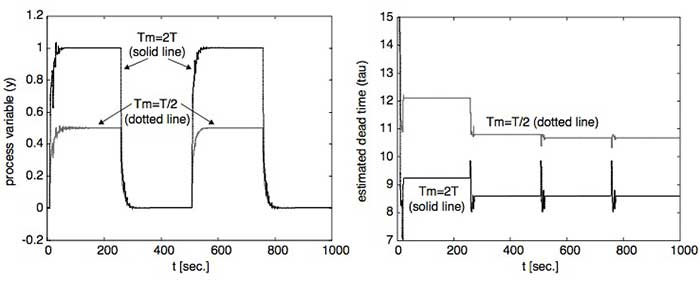Figure 8 Achievable Performances with a Modelling Error in the Dominant Lag Time Estimation (Solid Line: Tm=2T; Dotted Line: Tm=0.5T)

If the dominant lag time of the model is greater than that of the process (Tm>T), the transport dead time τ will be under- estimated (solid line, on the right side of Figure 8). This means that τmwill converge to a value lower than the real τ. On the contrary, if Tm>T, then the dead time will be super-estimated (dotted line, on the right side of Figure 8).

As seen in the left side of Figure 8, this is the best that the algorithm can do. However, the achievable performances are better than the standard Smith Predictor without any automatic time delay computation. For the purpose of presenting a clearly understood figure, the two setpoints have been scaled by a factor of 0.5.

CONCLUSIONS

In this paper the theory behind the control process affected by dead time through the Smith Predictor has been explained. Furthermore, considering the common FOPTD (First Order Plus Time Delay) process, it has been shown how easy it is to implement it with Yokogawa controller (YS170) and DCS (CENTUM CS1000/3000).

Since the dead time can change depending on the fluid speed in the pipelines, a new law for its automatic computation has been proposed for supporting the operators in the fine tuning of the algorithm. Thanks to the suggested method, the dead time can be quickly estimated through quite simple step changes in the manipulated variable (open loop approach) or even in the setpoint (closed loop approach). These step changes can be applied manually by the operator when it is realized that the process variable behaviour is not satisfactory. Step changes in the setpoint can also be part of a program which has to be run automatically when the measured variable is too unstable.

The algorithm works better if a good model of the process is available, but it works well even if the main lag time is only roughly estimated. Should the system under control be an integral process, additional Matausek-Micic feedback should also be incorporated into the control strategy.

Recently some other modifications of the Smith Predictor have been proposed (see bibliography). They are, however, not focused on algorithm tuning. In these proposed modifications, if the dead time of the model is wrong, the performance dramatically decreases (become unstable). On the other hand, the proposed method in this paper can improve performance in accommodating a wrong value of the dead time of the model (τm) due to bad initial tuning or even to changes in the working conditions in the process (i.e. a change of the flow rate in the pipelines).

REFERENCES

1. K. J. Astrom, Hang Lim, "A new Smith Predictor for controlling a process with an integrator and long dead-time", IEEE Trans. Autom. Contr., Vol. 39, pp. 343-345, Feb. 1994
2. T. Hagglund, "An industrial dead-time compensating PI controller", Cont. Eng. Pract., Vol. 4, No. 6, pp. 749-756, 1996
3. M. R. Matausek, A. D. Micic, "A modified Smith Predictor for controlling a process with an integrator and long dead- time", IEEE Transaction on Automatic Control, Vol. 41, No. 8, pp. 1199-1203, 1996
4. J. E. Normey-Rico, E. F. Camacho, "Robust tuning of dead- time compensator for process with an integrator and long dead time", IEEE Transaction on Automatic Control, Vol.44, No.8, pp-1597-1605, Aug.1999
5. Z. Palmor, "Stability properties of Smith dead time compensator controllers", International Journal of Control, Vol. 32, No. 6, pp.937-949, 1980
6. O. J. M. Smith, "A controller to overcome dead time", ISA Journal, Vol. 6, No. 2, pp. 28-33, 1959
7. F. G. Shinskey, "PID deadtime control of distributed process" Preprints IFAC Workshop on Digital Control – PID'00, Terrassa (E), 2000
8. M. Veronesi, "Regolazione PID. Fondamenti di teoria, algoritmi di taratura, applicazioni di controllo", Franco Angeli, in Italian 2002
9. M. Veronesi, A. Visioli, "Controllo di processi industriali affetti da ritardo", Atti del Convegno Automazione e processi decisionali, ANIPLA, in Italian, 2000

Related Products & Solutions

YS170 Single-loop Programmable Controller

The YS170 Single-loop Programmable Controller was discontinued on 1/01/2008. The YS1000 Series is a replacement product.

Distributed Control System (DCS)

Our distributed control system (DCS) enables automation and control of industrial processes and enhanced business performance. Over 10,000 plants entrust Yokogawa DCS to deliver their production goals.

Single Loop Controllers

Single loop controllers receive temperature, flow rate, pressure, and other types of measurement readings from sensors and send corresponding instructions to control elements such as valves in order to maintain these values within a desired range.

Top